# Selina Solutions Concise Maths Class 10 Chapter 10 Arithmetic Progression Exercise 10(F)

This exercise contains mixed problems based on all the important concepts of A.P. For acquiring a firm grip over solving problems of A.P, students can make use of the Selina Solutions for Class 10 Maths. The solutions are precise and are prepared in simple language to meet the needs of all students. Further, the solutions to this exercise are available in the Selina Solutions Concise Maths Class 10 Chapter 10 Arithmetic Progression Exercise 10(F), PDF in the links given below.

## Selina Solutions Concise Maths Class 10 Chapter 10 Arithmetic Progression Exercise 10(F) Download PDF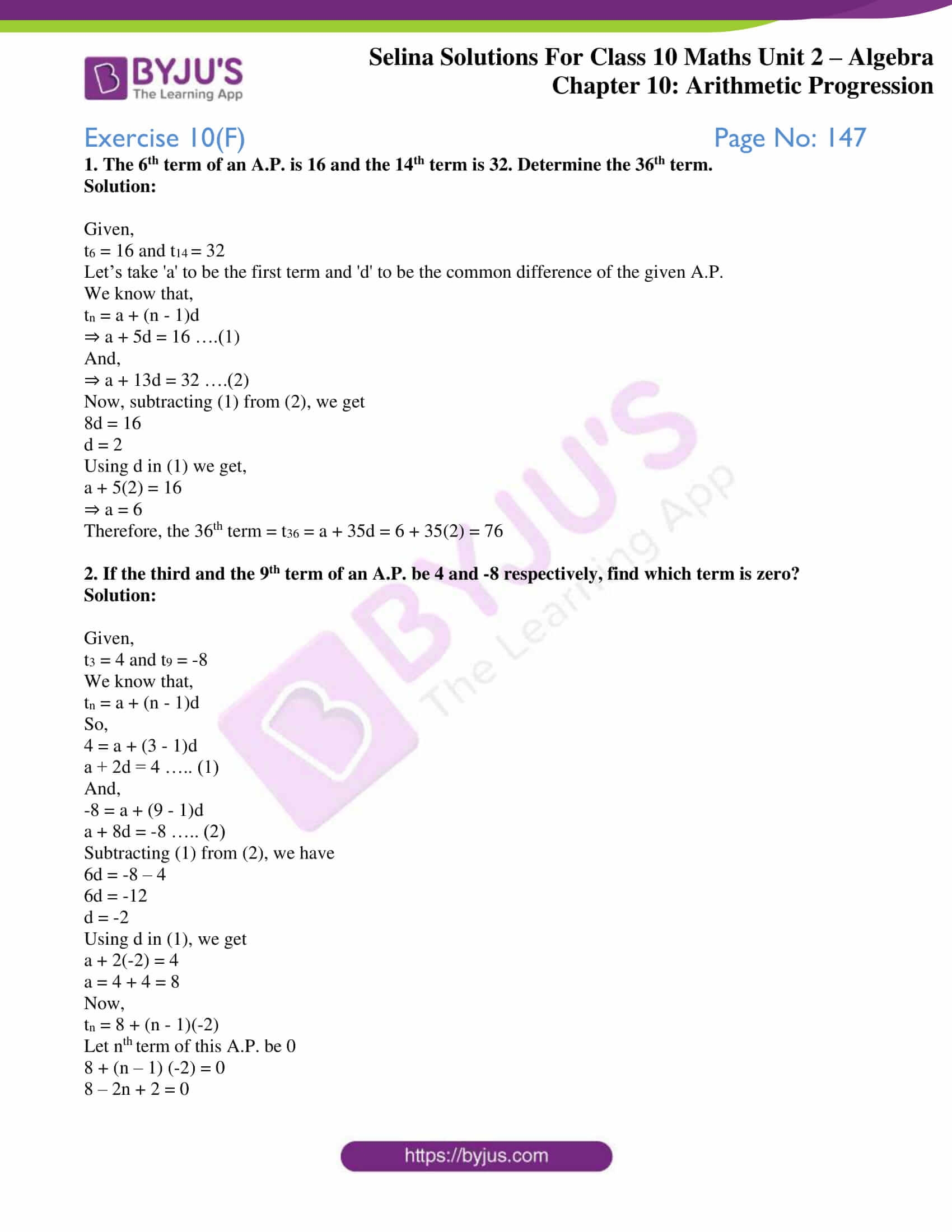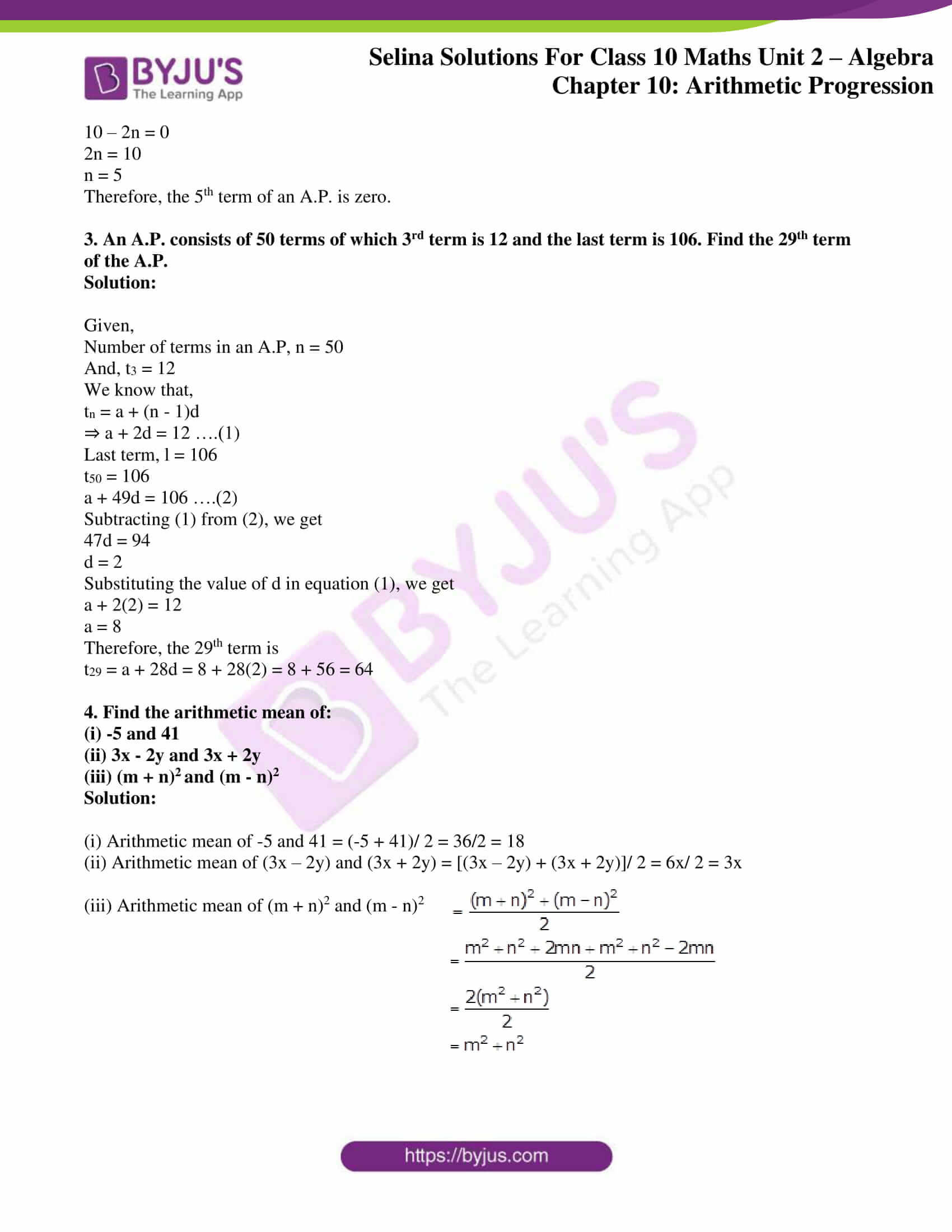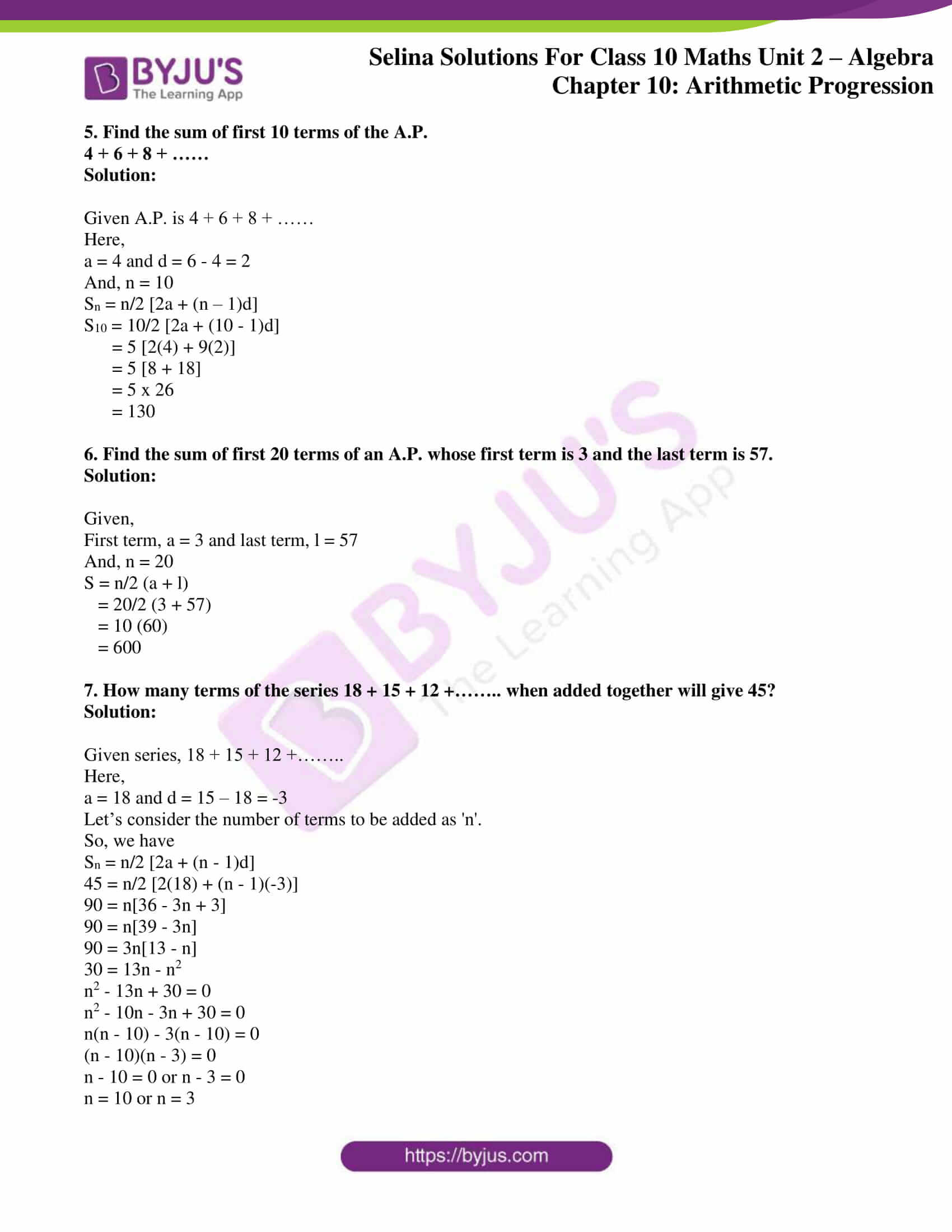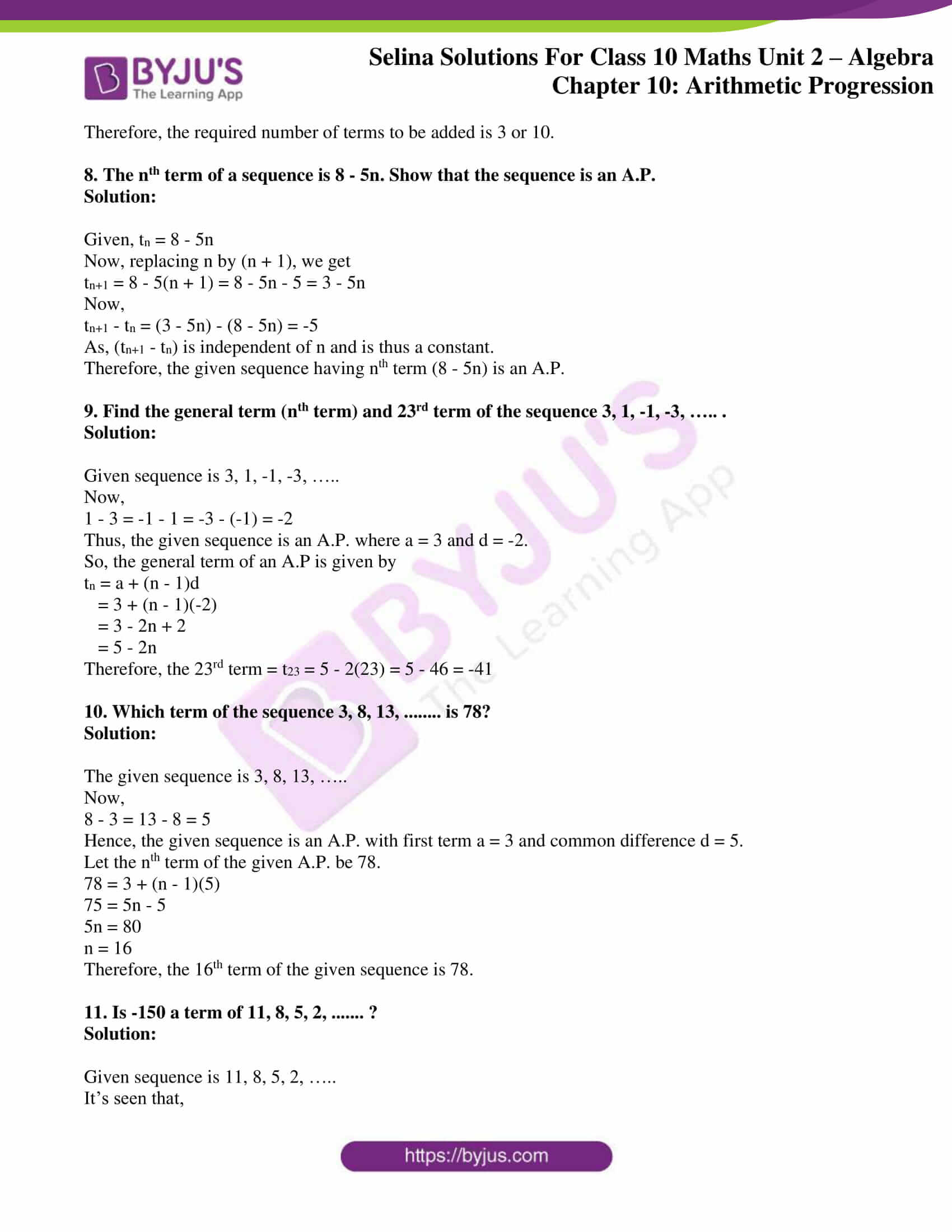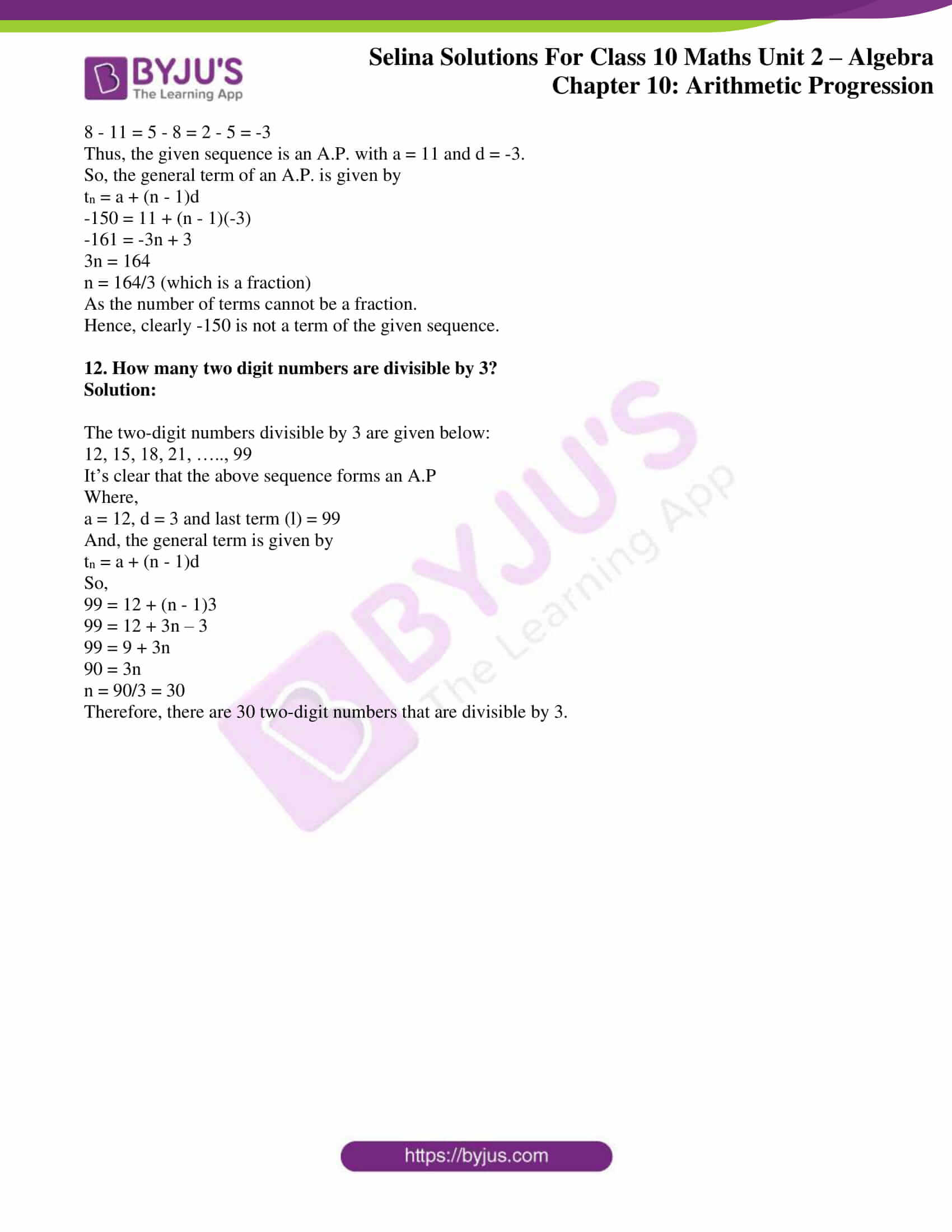### Access Selina Solutions Concise Maths Class 10 Chapter 10 Arithmetic Progression Exercise 10(F)

#### Exercise 10(F) Page No: 147

1. The 6th term of an A.P. is 16 and the 14th term is 32. Determine the 36th term.

Solution:

Given,

t6 = 16 and t14 = 32

Let’s take ‘a’ to be the first term and ‘d’ to be the common difference of the given A.P.

We know that,

tn = a + (n – 1)d

⇒ a + 5d = 16 ….(1)

And,

⇒ a + 13d = 32 ….(2)

Now, subtracting (1) from (2), we get

8d = 16

d = 2

Using d in (1) we get,

a + 5(2) = 16

⇒ a = 6

Therefore, the 36th term = t36 = a + 35d = 6 + 35(2) = 76

2. If the third and the 9th term of an A.P. be 4 and -8 respectively, find which term is zero?

Solution:

Given,

t3 = 4 and t9 = -8

We know that,

tn = a + (n – 1)d

So,

4 = a + (3 – 1)d

a + 2d = 4 ….. (1)

And,

-8 = a + (9 – 1)d

a + 8d = -8 ….. (2)

Subtracting (1) from (2), we have

6d = -8 – 4

6d = -12

d = -2

Using d in (1), we get

a + 2(-2) = 4

a = 4 + 4 = 8

Now,

tn = 8 + (n – 1)(-2)

Let nth term of this A.P. be 0

8 + (n – 1) (-2) = 0

8 – 2n + 2 = 0

10 – 2n = 0

2n = 10

n = 5

Therefore, the 5th term of an A.P. is zero.

3. An A.P. consists of 50 terms of which 3rd term is 12 and the last term is 106. Find the 29th term of the A.P.

Solution:

Given,

Number of terms in an A.P, n = 50

And, t3 = 12

We know that,

tn = a + (n – 1)d

⇒ a + 2d = 12 ….(1)

Last term, l = 106

t50 = 106

a + 49d = 106 ….(2)

Subtracting (1) from (2), we get

47d = 94

d = 2

Substituting the value of d in equation (1), we get

a + 2(2) = 12

a = 8

Therefore, the 29th term is

t29 = a + 28d = 8 + 28(2) = 8 + 56 = 64

4. Find the arithmetic mean of:

(i) -5 and 41

(ii) 3x – 2y and 3x + 2y

(iii) (m + n)and (m – n)2

Solution:

(i) Arithmetic mean of -5 and 41 = (-5 + 41)/ 2 = 36/2 = 18

(ii) Arithmetic mean of (3x – 2y) and (3x + 2y) = [(3x – 2y) + (3x + 2y)]/ 2 = 6x/ 2 = 3x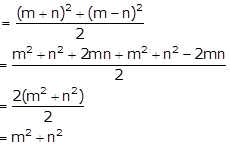(iii) Arithmetic mean of (m + n)2 and (m – n)2

5. Find the sum of first 10 terms of the A.P.

4 + 6 + 8 + ……

Solution:

Given A.P. is 4 + 6 + 8 + ……

Here,

a = 4 and d = 6 – 4 = 2

And, n = 10

Sn = n/2 [2a + (n – 1)d]

S10 = 10/2 [2a + (10 – 1)d]

= 5 [2(4) + 9(2)]

= 5 [8 + 18]

= 5 x 26

= 130

6. Find the sum of first 20 terms of an A.P. whose first term is 3 and the last term is 57.

Solution:

Given,

First term, a = 3 and last term, l = 57

And, n = 20

S = n/2 (a + l)

= 20/2 (3 + 57)

= 10 (60)

= 600

7. How many terms of the series 18 + 15 + 12 +…….. when added together will give 45?

Solution:

Given series, 18 + 15 + 12 +……..

Here,

a = 18 and d = 15 – 18 = -3

Let’s consider the number of terms to be added as ‘n’.

So, we have

Sn = n/2 [2a + (n – 1)d]

45 = n/2 [2(18) + (n – 1)(-3)]

90 = n[36 – 3n + 3]

90 = n[39 – 3n]

90 = 3n[13 – n]

30 = 13n – n2

n2 – 13n + 30 = 0

n2 – 10n – 3n + 30 = 0

n(n – 10) – 3(n – 10) = 0

(n – 10)(n – 3) = 0

n – 10 = 0 or n – 3 = 0

n = 10 or n = 3

Therefore, the required number of terms to be added is 3 or 10.

8. The nth term of a sequence is 8 – 5n. Show that the sequence is an A.P.

Solution:

Given, tn = 8 – 5n

Now, replacing n by (n + 1), we get

tn+1 = 8 – 5(n + 1) = 8 – 5n – 5 = 3 – 5n

Now,

tn+1 – tn = (3 – 5n) – (8 – 5n) = -5

As, (tn+1 – tn) is independent of n and is thus a constant.

Therefore, the given sequence having nth term (8 – 5n) is an A.P.

9. Find the general term (nth term) and 23rd term of the sequence 3, 1, -1, -3, ….. .

Solution:

Given sequence is 3, 1, -1, -3, …..

Now,

1 – 3 = -1 – 1 = -3 – (-1) = -2

Thus, the given sequence is an A.P. where a = 3 and d = -2.

So, the general term of an A.P is given by

tn = a + (n – 1)d

= 3 + (n – 1)(-2)

= 3 – 2n + 2

= 5 – 2n

Therefore, the 23rd term = t23 = 5 – 2(23) = 5 – 46 = -41

10. Which term of the sequence 3, 8, 13, …….. is 78?

Solution:

The given sequence is 3, 8, 13, …..

Now,

8 – 3 = 13 – 8 = 5

Hence, the given sequence is an A.P. with first term a = 3 and common difference d = 5.

Let the nth term of the given A.P. be 78.

78 = 3 + (n – 1)(5)

75 = 5n – 5

5n = 80

n = 16

Therefore, the 16th term of the given sequence is 78.

11. Is -150 a term of 11, 8, 5, 2, ……. ?

Solution:

Given sequence is 11, 8, 5, 2, …..

It’s seen that,

8 – 11 = 5 – 8 = 2 – 5 = -3

Thus, the given sequence is an A.P. with a = 11 and d = -3.

So, the general term of an A.P. is given by

tn = a + (n – 1)d

-150 = 11 + (n – 1)(-3)

-161 = -3n + 3

3n = 164

n = 164/3 (which is a fraction)

As the number of terms cannot be a fraction.

Hence, clearly -150 is not a term of the given sequence.

12. How many two digit numbers are divisible by 3?

Solution:

The two-digit numbers divisible by 3 are given below:

12, 15, 18, 21, ….., 99

It’s clear that the above sequence forms an A.P

Where,

a = 12, d = 3 and last term (l) = 99

And, the general term is given by

tn = a + (n – 1)d

So,

99 = 12 + (n – 1)3

99 = 12 + 3n – 3

99 = 9 + 3n

90 = 3n

n = 90/3 = 30

Therefore, there are 30 two-digit numbers that are divisible by 3.

### Access other exercises of Selina Solutions Concise Maths Class 10 Chapter 10 Arithmetic Progression

Exercise 10(A) Solutions

Exercise 10(B) Solutions

Exercise 10(C) Solutions

Exercise 10(D) Solutions

Exercise 10(E) Solutions